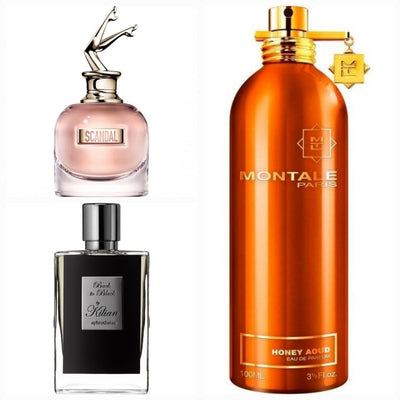Browse
Shopping Cart
– 20%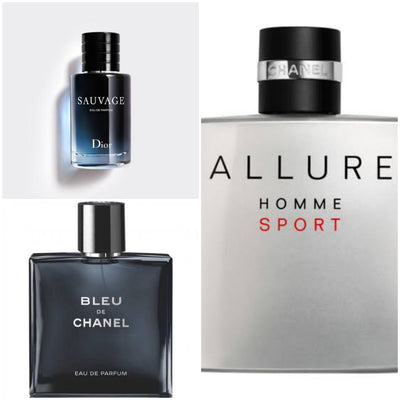Rs. 2,499.00 Rs. 1,999.00
– 37%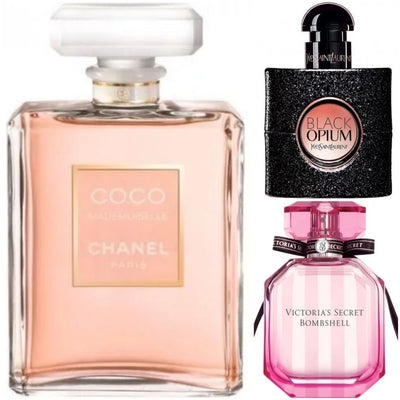Rs. 2,999.00 Rs. 1,899.00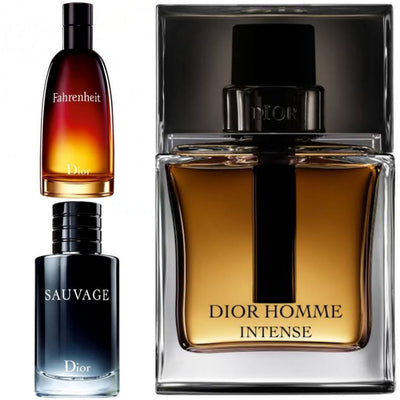Rs. 1,999.00
– 37%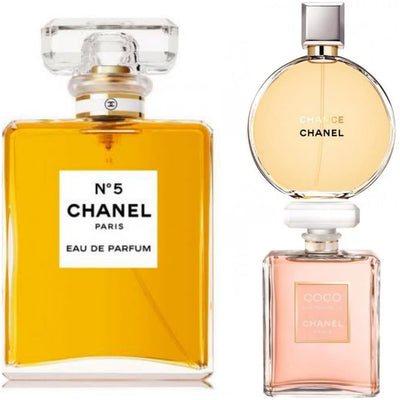Rs. 2,999.00 Rs. 1,899.00
– 7%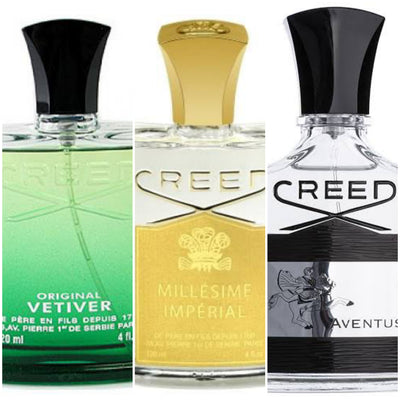Rs. 2,999.00 Rs. 2,799.00
– 7%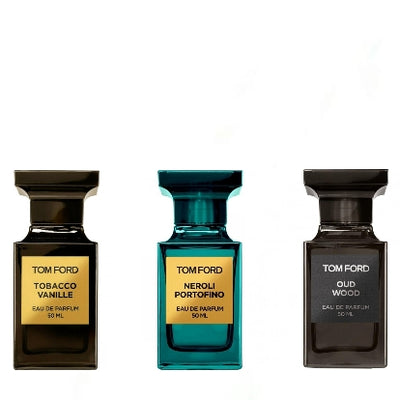Rs. 2,999.00 Rs. 2,799.00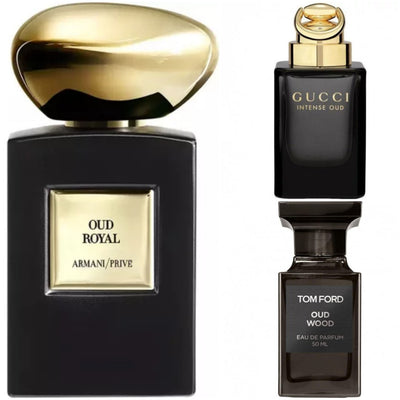Rs. 2,550.00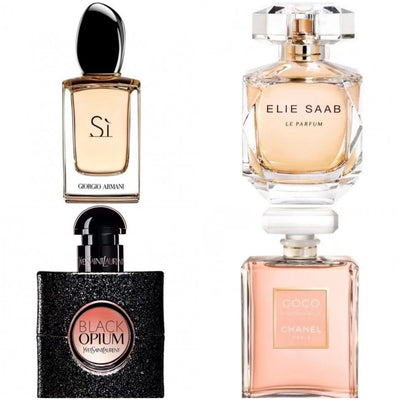Rs. 2,499.00
– 53%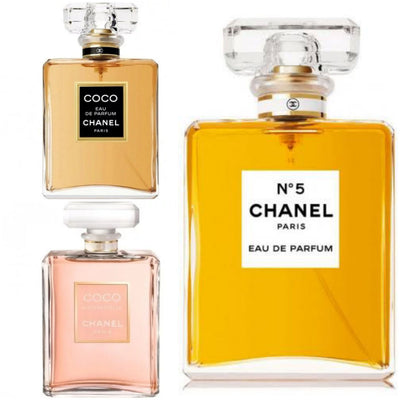Rs. 3,999.00 Rs. 1,899.00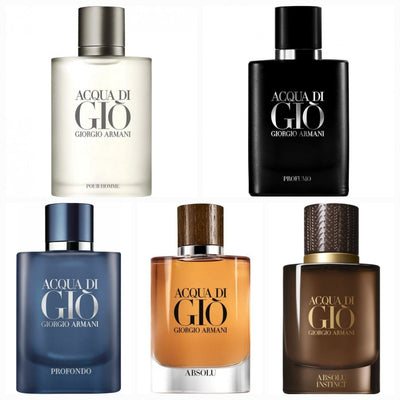Rs. 2,999.00
– 44%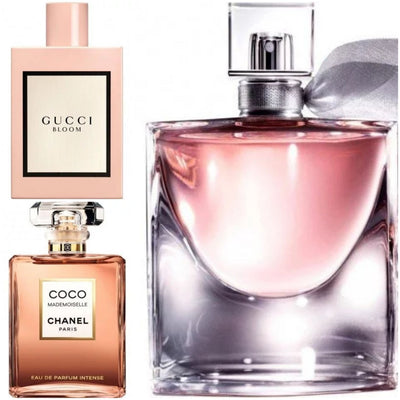Rs. 3,999.00 Rs. 2,250.00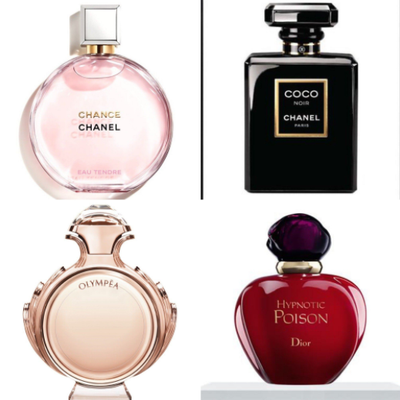Rs. 2,799.00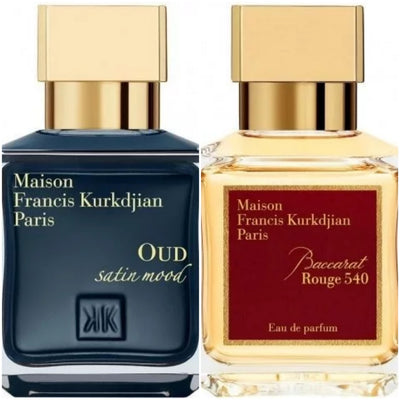Rs. 1,999.00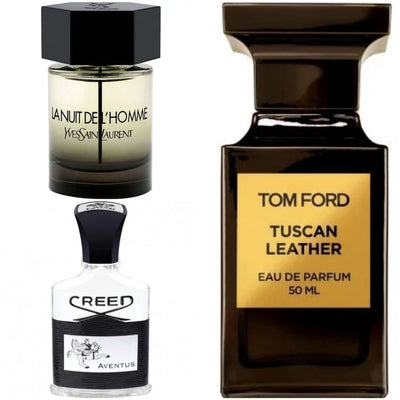Rs. 2,799.00
– 7%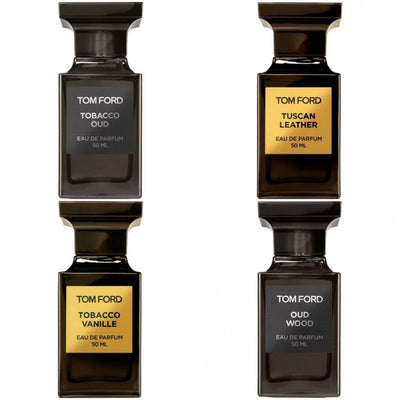Rs. 6,999.00 Rs. 6,499.00
– 11%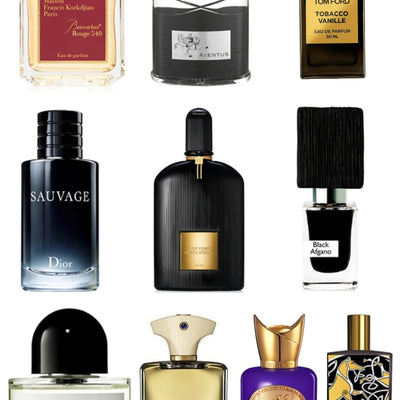Rs. 5,000.00 Rs. 4,449.00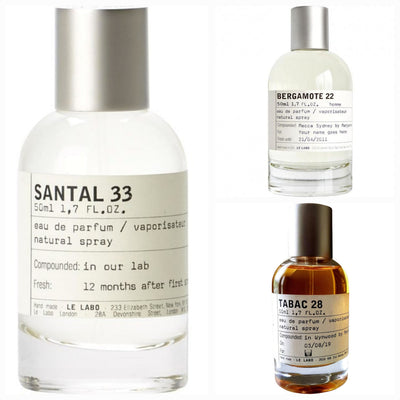Rs. 2,400.00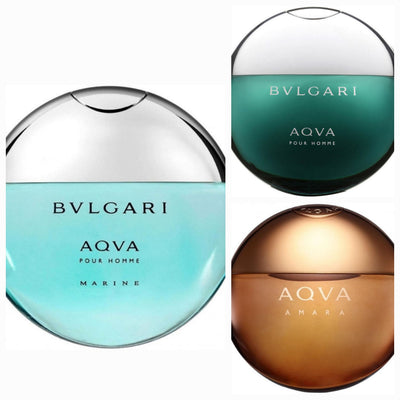Rs. 1,650.00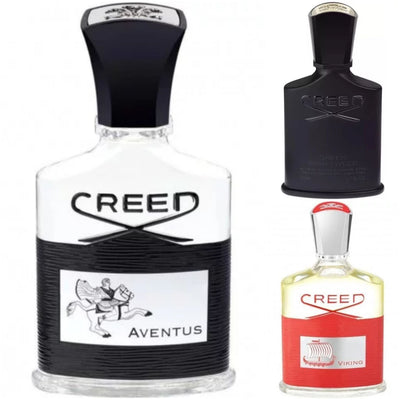Rs. 2,799.00
– 7%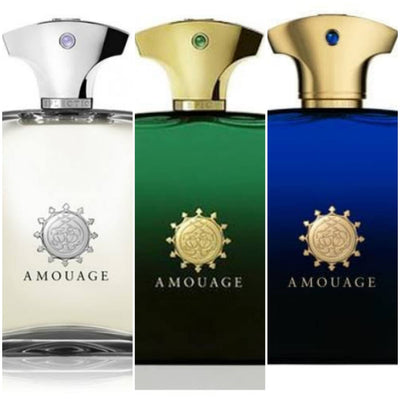Rs. 2,999.00 Rs. 2,799.00

– 20%Rs. 2,499.00 Rs. 1,999.00
– 37%Rs. 2,999.00 Rs. 1,899.00Rs. 1,999.00
– 37%Rs. 2,999.00 Rs. 1,899.00
– 7%Rs. 2,999.00 Rs. 2,799.00
– 7%Rs. 2,999.00 Rs. 2,799.00Rs. 2,550.00Rs. 2,499.00
– 53%Rs. 3,999.00 Rs. 1,899.00Rs. 2,999.00
– 44%Rs. 3,999.00 Rs. 2,250.00Rs. 2,799.00Rs. 1,999.00Rs. 2,799.00
– 7%Rs. 6,999.00 Rs. 6,499.00
– 11%Rs. 5,000.00 Rs. 4,449.00Rs. 2,400.00Rs. 1,650.00Rs. 2,799.00
– 7%Rs. 2,999.00 Rs. 2,799.00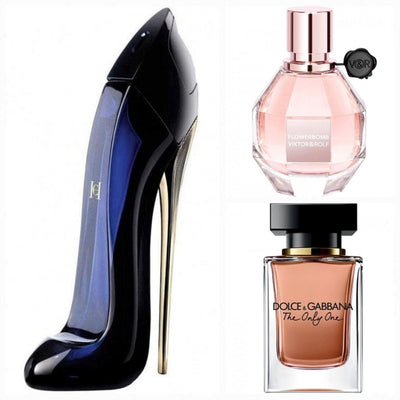Rs. 2,200.00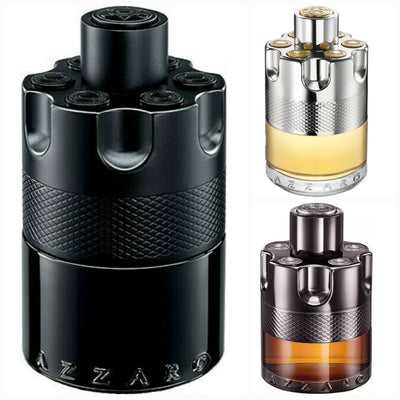Rs. 2,000.00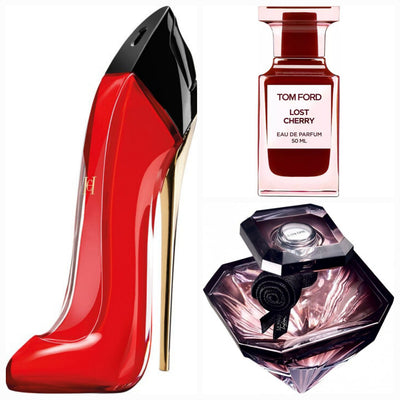Rs. 2,500.00Rs. 2,999.00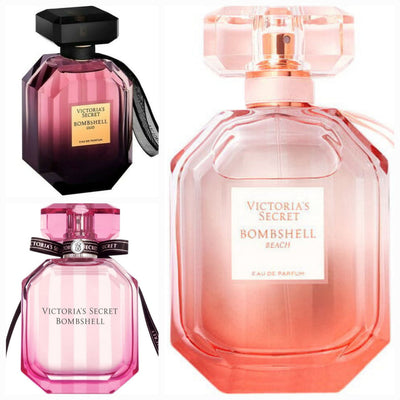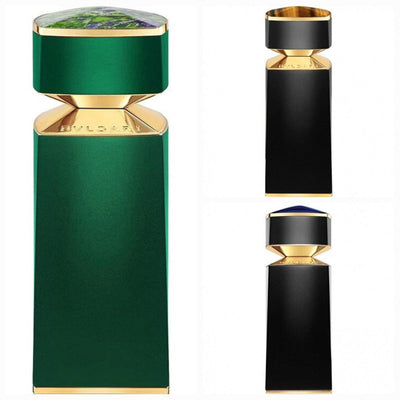Rs. 2,400.00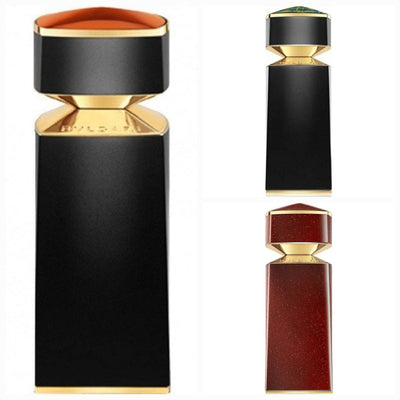Rs. 2,400.00